Make it to the Right and Larger Audience

Blog

Questions are in the first page and answers are in the second page.

1. Which of the following core areas will produce the smallest die size?
1. Nop-flipped and separated rows
2. Flipped and abutted rows
3. Flipped and separated rows
4. Non-flipped and abutted rows

1. Refer to layout A & B, which of the following is true: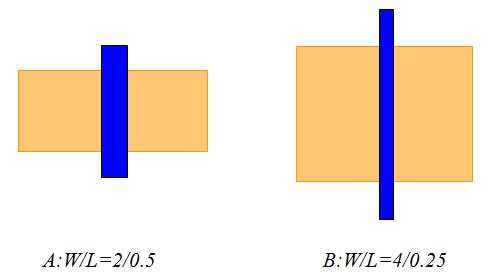1. B has the same area as A and double the drive.
2. B has double the area of A and half the drive.
3. B has the same area as A and the same drive.
4. B has 4X the area as A and the same drive.
5. B has 4X the drive as A and the same area.

1. which of the following is correct?
1. Slack=Data arrival time － Data Required time
2. Slack=Data Required time － Data arrival time
3. Slack=Clock Arrival time － Data arrival time
4. Slack=Clock Required time － Data Required time

1. In the following figure, what is the total number of possible data arrival times for setup?
1. 1 ns
2. 2 ns
3. 3 ns
4. 4 ns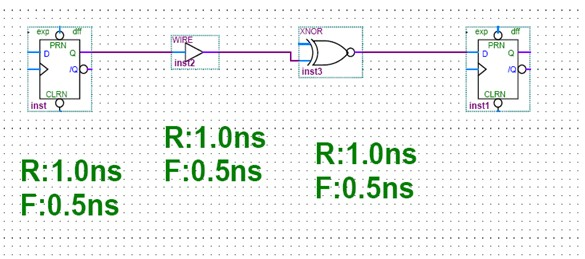1. Which will always result in the worst slack for setup time?
1. The longest data arrival time.
2. The shortest data arrival time.
3. It is unclear as the slack is a function of data arrival time as well as data required time.

1. n-device is faster than p-device because
1. Hole mobility is greater than electron mobility.
2. Electron mobility is greater than hole mobility.
3. The P threshold is greater than N threshold.
4. The N threshold is greater than P threshold.
5. None of the above.

1. For a 25 MHz processor, what is the time taken by the instruction which needs 3 clock cycle
1. 120 nano secs
2. 120 micro secs
3. 75 nano secs
4. 75 micro secs

1. Which one is correct statement?
1. RTL models can be simulated only on cycle based simulator
2. Event driven simulators can simulate both synchronous and asynchronous designs
3. Multi clock designs can not be simulated with Event driven simulators
4. b and c

1. Formal analysis means:
1. Verifying designs with assertions using simulators
2. Verifying designs only with assertions statically
3. Analyzing functional coverage generated from the assertion IPs
4. a and c

1. Which language supports object oriented programming and good for RTL verification?
1. C++
2. Verilog with VPI
3. System Verilog
4. b and c

1. What is the difference between logic and bit types?
1. logic is 2 state, bit is 4 state
2. There is no difference
3. logic is 4 state, bit is 2 state
4. none of the above

1. through clocking blocks
2. Through cross module reference
3. Through modports
4. None of the above

1. How do I create a variable shared by all objects of a class, but not make a global?
1. declare it as automatic
2. declare it in the top scope
3. declare it as shared
4. declare it as static

1. How do I share code between classes?
1. Instantiate a class within another class
2. Inherit from one class to another (inheritance/derivation)
3. all of the above
4. None of the above

1. What is the final value of t1.data and t2.data in this code?

class Thing;

int data;

endclass
Thing t1, t2;

initial begin

t1 = new();

t1.data = 1;

t2.data = 2;

t2 = t1;

t1.data = 5;

end

1. t1.data = 5, t2.data = 5
2. t2 gets used before new() is called. Null object access!
3. t1.data = 5, t2.data =2;
4. None of the above

1. Write a constraint for the variable Q that picks a value of 0, for 75% of the time and 1~3 for the rest?
1. constraint c {Q dist {0 := 75, [1:3] := 25} ; }
2. constraint c { Q < 4; Q >= 0; }
3. constraint c { Q < 4; Q >= 0; Q%2 dist {0 := 75, 1:= 25}; }
4. constraint c { Q < 4; Q >= 0; Q%3 dist {0 := 25, 1:= 25, 2:=25, 3:= 75 }; }

1. What are the possible values that x and y will take when the following class is randomized

class E;    rand bit [15:0] x, y;    constraint size_cons {                           foreach (x){                            x > 0; x < y;                         foreach (y)                             y inside {[1:9]};                       }} endclass

1. x in [0,9], y in [0,9]
2. x in [1,9], y in [1,9]
3. x in [1,9], y in [2,10]
4. x in [1,8], y in [2,9]

1. What is the final value of t1.data and t2.data in this code?

class Thing;

int data;

endclass

Thing t1, t2;

initial begin

t1 = new();

t1.data = 1;

t2.data = 2;

t2 = t1;

t1.data = 5;

end

1. t1.data = 5, t2.data = 5
2. t2 gets used before new() is called. Null object access!
3. t1.data = 5, t2.data =2;
4. None of the above

1. The new intern Smart Assert can not write simple assertions. He wrote:

( a ##1 (!b[*0:\$] ##1 b)[*3:5] ## 1C )

While the assertion works correctly, can you help him write it in a simplified form? Please choose one correct answer from A), B), C) and D):

A)property abc_sequence_with_!b_random_space_between;

@(posedge clk) \$rose(a)##1 b|-> b[*3:5] ##1c;

endproperty: abc_sequence_with_!b_random_space_between;

B)property abc_sequence_with_!b_random_space_between;

@(posedge clk) a##1 b[=3:5] ##1 c;

endproperty: abc_sequence_with_!b_random_space_between;

C)property abc_sequence_with_!b_random_space_between;

@(posedge clk) a##1 !b[*0:\$] ##1 b[*3:5] ##1 c;

endproperty: abc_sequence_with_!b_random_space_between;

D)property abc_sequence_with_!b_random_space_between;

@(posedge clk) a##1 b[->3:5] ##1 c;

endproperty: acb_sequence_with_!b_random_space­_between;

1. Consider a bus protocol that includes the property “a new bus cycle may not start for 2 clock cycles after an abort cycle occurs.” This property could be coded as below. Please choose one correct answer from A), B), C) and D).

A）property wait_after_abort;

@(posedge clk) wait（abort_cycle） ##2 !cycle_start;

endproperty: wait_after_abort ;

B）property wait_after_abort;

@(posedge clk) while (abort_cycle) ##2 Not(cycle_start)  ;

endproperty: wait_after_abort

C）property wait_after_abort;

@(posedge clk) abort_cycle |=> !cycle_start[*2];

endproperty: wait_after_abort

D）property wait_after_abort;

@(posedge clk) abort_cycle |-> Not(##2 cycle_start);

endproperty: wait_after_abort

1. Consider the following logic expressions for f and g :

ƒ = x1++x1x2x4x5+x3+ x1x3x5 +x4+ x1x2x3x4

g =x3++ x1x3x4+ x1x4+ x1 x3x4x5++ x1x2x4x5

Prove or disprove that ƒ = g. Steps of logic simplification must be provided with the name of theorems used, or else no marks will be given.

1. In standard cell technology, circuits are built by interconnecting building-block cells that implement simple functions, like basic logic gates. A commonly used type of standard cells is And-Or-Invert (AOI), which can be efficiently built as CMOS complex gates. Consider the AOI cell shown in the following Figure. This cell implements the function f =. Derive the CMOS complex gate that implements this cell.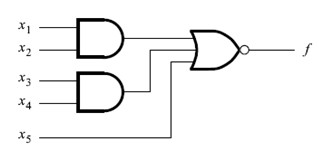1. Implement the following function:

f (w1,w2,w3,w4,w5) = + w1w2 + w1w3 + w1w4 + w3w4w5

by using a 4-to-1 multiplexer and as few other gates as possible. Assume that only the

uncomplemented inputs w1, w2, w3,w4 and w5 are available.

1. A sequential circuit has two inputs, w1 and w2, and an output , z .Its function is to compare the input sequences on the two inputs . If w1 = w2 during any four consecutive clock cycles, the circuit produces z = 1; otherwise , z = 0. For example

w1 : 0110111000110

w2 : 1110101000111

z : 0000100001110

Derive a suitable circuit . Write the Verilog or VHDL codes for the FSM which describes the circult.

1. For the circuit of Figure below, show the tests that can detect each of the faults:

w1/0, w4/1, g/0, c/1.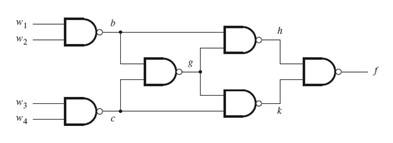1. A circuit has an input w and an output z. A sequence of pulses is applied on input w. The output has to replicate every third pulse, as illustrated in Figure low. Design a suitable circuit which is composed only by gates with delay, no flip-flops are allowed in the circuit.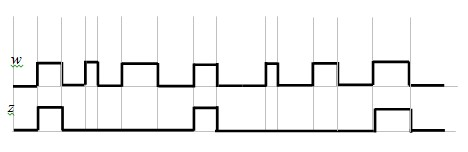1. Derive the state table for the circuit below. What sequence of input values on wire

w is detected by this circuit?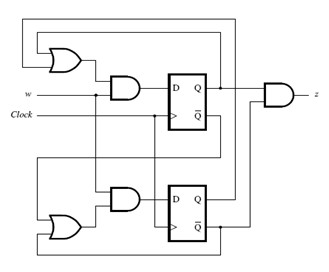1. Assume a circuit that can convert the serial bit flow, sdata, into an output of one hot bit parallel data, pdata[7:0] . The protocol for the serial bit flow, sdata, is that LSB is sent first, and MSB last. That sdata changes from 1 to 0 when sclk is high, means that the sdata is ready to send. That sdata changes from 0 to1, when sclk is high, means that the sdata sending has been finished. And sdata bits can only change its 1/0 when sclk is low. See the waveform below. Write the synthesizable Verilog or VHDL model for the circuit with the above behave. No other signals can be used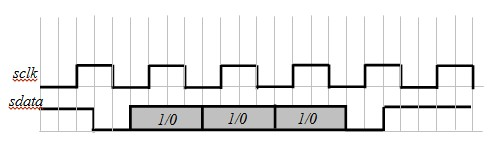1. Using Verilog or VHDL to write the test-bench for fully testing and verify the above circuit.Staff Hardware Engineer
Author brief is empty
Groups:

Tags: